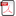### A Conditional Mean Square Estimate for the Solution of a SDE

•  Andriy Yurachkivsky

#### Abstract

Let $\bF=\left(\cF(t), \:t\in \bR_+\right)$ be a filtration on some probability space, and $X$ be the strong solution of the equation $X(t)=\X+\int_0^tQ(s,X(s))\rd\iota(s)+\int_0^t\sigma(s,X(s-)) \rd Y(s),$ where $\X$ is an $\cF(0)$-measurable $\bR^d$-valued random variable, $\iota$ is a continuous increasing process with $\cF(0)$-measurable values at all times, $Y$ is an $\bR^m$-valued locally square integrable martingale with respect to $\bF$ subjected to some mild additional demands, $Q$ and $\sigma$ are continuous in $x\in\bR^d$ random functions on $\bR_+\times\bR^d$ (the former $\bR^d$-valued and $\bF$-progressive in $(\omega,t)\in\Omega\times\bR_+$, the latter ($d\times m)$-matrix-valued and $\bF$-predictable). Suppose also that there exists an $\cF(0)\otimes\cB_+$-measurable in $(\omega,t)$ nonnegative random process $\psi$ such that, for all $t,x$, $x^\top Q(t,x) \le-\psi(t)|x|^2$ and $\int_0^t\psi(s)\rd\iota(s)<\infty.$ Under these assumptions, $\E(|X(t)|^2|\cF(0)$ is evaluated from above.

• Full Text:PDF
• DOI:10.5539/ijsp.v3n4p1This work is licensed under a Creative Commons Attribution 4.0 License.
• ISSN(Print): 1927-7032
• ISSN(Online): 1927-7040
• Started: 2012
• Frequency: bimonthly

### Journal Metrics

• h-index (December 2020): 18
• i10-index (December 2020): 36
• h5-index (December 2020): N/A
• h5-median(December 2020): N/A